# NCERT Solutions for Class 10 Maths Chapter 3 – Pair of Linear Equations in Two Variables

## Exercise 3.1 Page: 44

1. Aftab tells his daughter, “Seven years ago, I was seven times as old as you were then. Also, three years from now, I shall be three times as old as you will be.” (Isn’t this interesting?) Represent this situation algebraically and graphically.

Solutions:Let the present age of Aftab be ‘x’.

And, the present age of his daughter be ‘y’.

Now, we can write, seven years ago,

Age of Aftab = x-7

Age of his daughter = y-7

According to the question,

x−7 = 7(y−7)

⇒x−7 = 7y−49

⇒x−7y = −42         ………………………(i)

Also, three years from now or after three years,

Age of Aftab will become = x+3.

Age of his daughter will become = y+3

According to the situation given,

x+3 = 3(y+3)

⇒x+3 = 3y+9

⇒x−3y = 6       …………..…………………(ii)

Subtracting equation (i) from equation (ii) we have

(x−3y)−(x−7y) = 6−(−42)

⇒−3y+7y = 6+42

⇒4y = 48

⇒y = 12

The algebraic equation is represented by

x−7y = −42

x−3y = 6

For, x−7y = −42 or x = −42+7y

The solution table is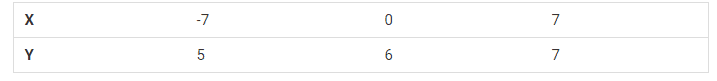For,  x−3y = 6   or     x = 6+3y

The solution table is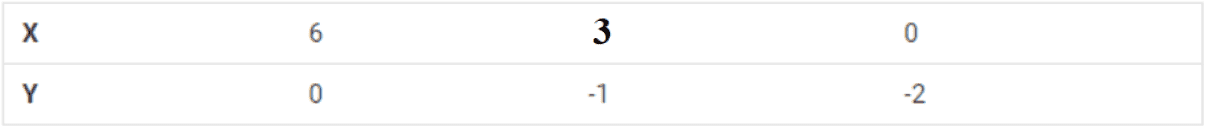The graphical representation is: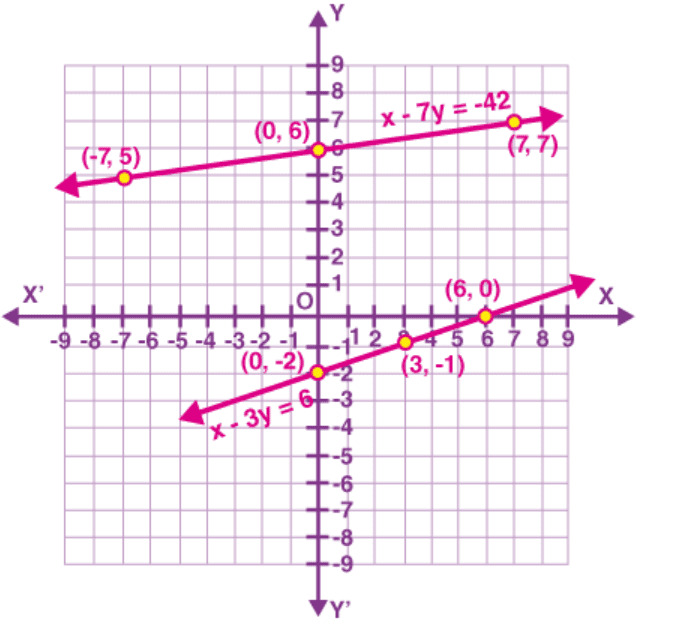2. The coach of a cricket team buys 3 bats and 6 balls for Rs.3900. Later, she buys another bat and 3 more balls of the same kind for Rs.1300. Represent this situation algebraically and geometrically.

Solutions:Let us assume that the cost of a bat be ‘Rs x’

And,the cost of a ball be ‘Rs y’

According to the question, the algebraic representation is

3x+6y = 3900

And x+3y = 1300

For, 3x+6y = 3900

Or x = (3900-6y)/3

The solution table is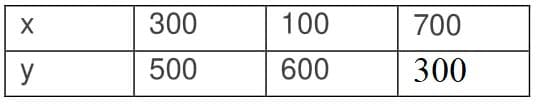For, x+3y = 1300

Or x = 1300-3y

The solution table is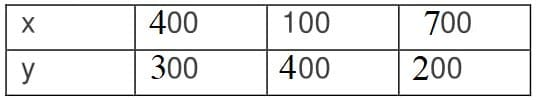The graphical representation is as follows.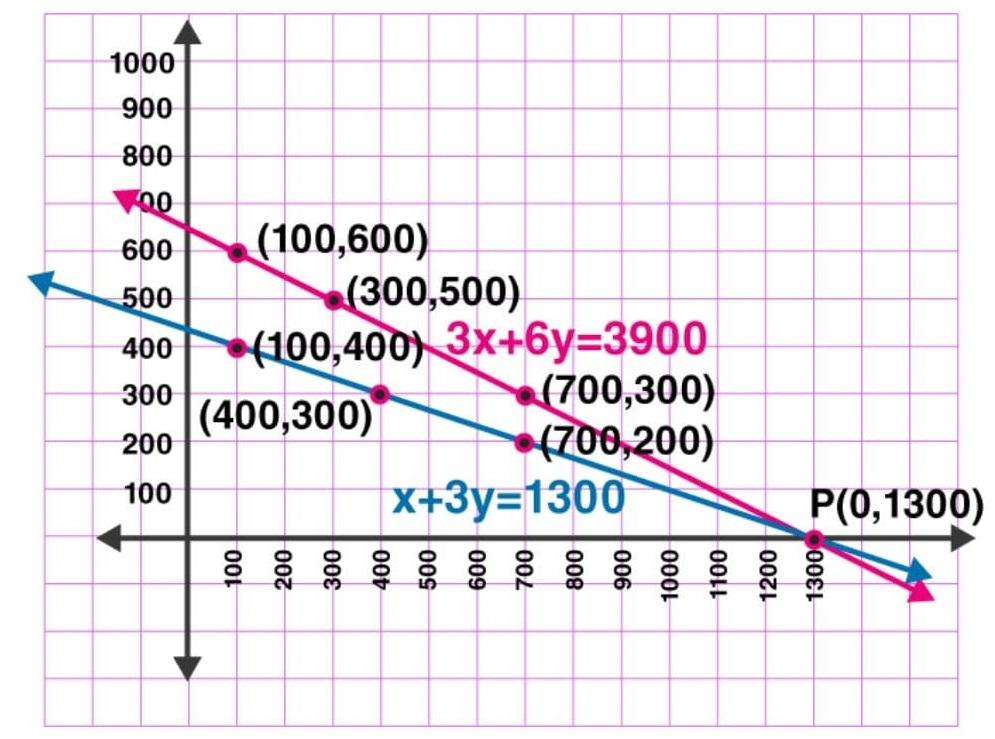3. The cost of 2 kg of apples and 1kg of grapes on a day was found to be Rs.160. After a month, the cost of 4 kg of apples and 2 kg of grapes is Rs.300. Represent the situation algebraically and geometrically.

Solutions:Let the cost of 1 kg of apples be ‘Rs. x’

And, cost of 1 kg of grapes be ‘Rs. y’

According to the question, the algebraic representation is

2x+y = 160

And 4x+2y = 300

For, 2x+y = 160 or y = 160−2x, the solution table is;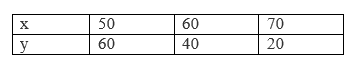For 4x+2y = 300 or y = (300-4x)/2, the solution table is;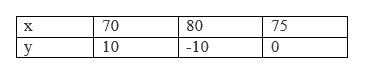The graphical representation is as follows;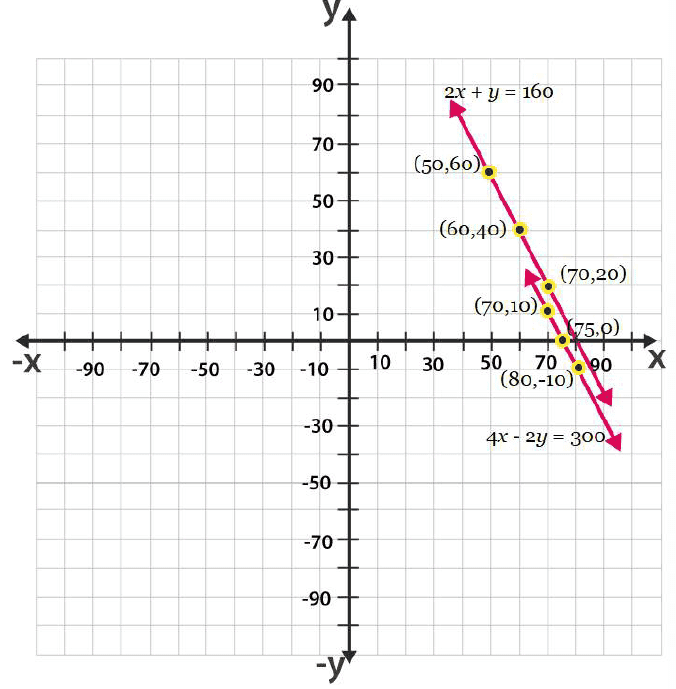## Exercise 3.2 Page: 49

1. Form the pair of linear equations in the following problems, and find their solutions graphically.

(i) 10 students of Class X took part in a Mathematics quiz. If the number of girls is 4 more than the number of boys, find the number of boys and girls who took part in the quiz.

(ii) 5 pencils and 7 pens together cost 50, whereas 7 pencils and 5 pens together cost 46. Find the cost of one pencil and that of one pen.

Solution:

(i)Let there are x number of girls and y number of boys. As per the given question, the algebraic expression can be represented as follows.

x +y = 10

x– y = 4

Now, for x+y = 10 or x = 10−y, the solutions are;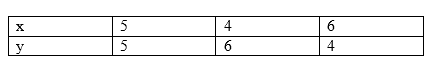For x – y = 4 or x = 4 + y, the solutions are;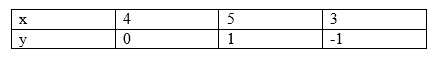The graphical representation is as follows;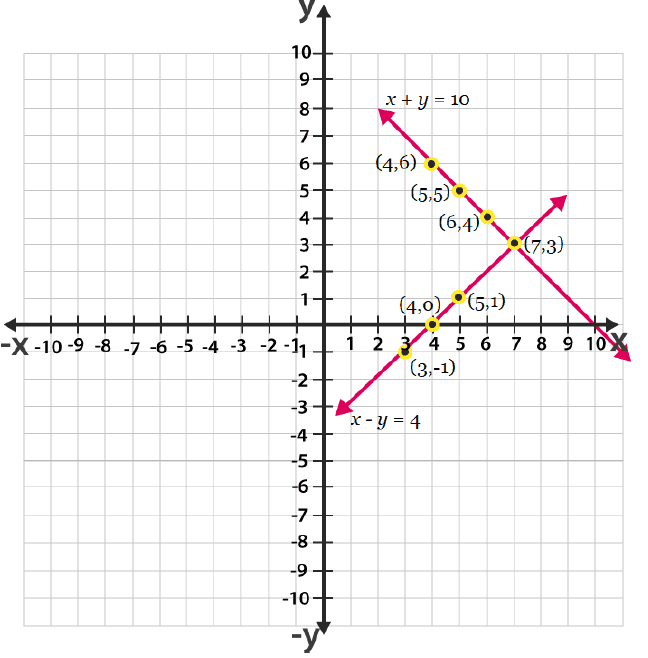From the graph, it can be seen that the given lines cross each other at point (7, 3). Therefore, there are 7 girls and 3 boys in the class.

(ii)Let 1 pencil costs Rs.x and 1 pen costs Rs.y.

According to the question, the algebraic expression cab be represented as;

5x + 7y = 50

7x + 5y = 46

For, 5x + 7y = 50 or  x = (50-7y)/5, the solutions are;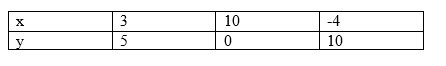For 7x + 5y = 46 or x = (46-5y)/7, the solutions are;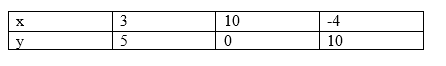Hence, the graphical representation is as follows;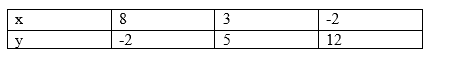From the graph, it is can be seen that the given lines cross each other at point (3, 5).

So, the cost of a pencil is 3/- and cost of a pen is 5/-.

2. On comparing the ratios a1/a2 , b1/b2 , c1/c2 find out whether the lines representing the following pairs of linear equations intersect at a point, are parallel or coincident:

(i) 5x – 4y + 8 = 0

7x + 6y – 9 = 0

(ii) 9x + 3y + 12 = 0

18x + 6y + 24 = 0

(iii) 6x – 3y + 10 = 0

2x – y + 9 = 0

Solutions:

(i) Given expressions;

5x−4y+8 = 0

7x+6y−9 = 0

Comparing these equations with a1x+b1y+c1 = 0

And a2x+b2y+c2 = 0

We get,

a1 = 5, b1 = -4, c1 = 8

a2 = 7, b2 = 6, c2 = -9

(a1/a2) = 5/7

(b1/b2) = -4/6 = -2/3

(c1/c2) = 8/-9

Since, (a1/a2) ≠ (b1/b2)

So, the pairs of equations given in the question have a unique solution and the lines cross each other at exactly one point.

(ii) Given expressions;

9x + 3y + 12 = 0

18x + 6y + 24 = 0

Comparing these equations with a1x+b1y+c1 = 0

And a2x+b2y+c2 = 0

We get,

a1 = 9, b1 = 3, c1 = 12

a2 = 18, b2 = 6, c2 = 24

(a1/a2) = 9/18 = 1/2

(b1/b2) = 3/6 = 1/2

(c1/c2) = 12/24 = 1/2

Since (a1/a2) = (b1/b2) = (c1/c2)

So, the pairs of equations given in the question have infinite possible solutions and the lines are coincident.

(iii) Given Expressions;

6x – 3y + 10 = 0

2x – y + 9 = 0

Comparing these equations with a1x+b1y+c1 = 0

And a2x+b2y+c2 = 0

We get,

a1 = 6, b1 = -3, c1 = 10

a2 = 2, b2 = -1, c2 = 9

(a1/a2) = 6/2 = 3/1

(b1/b2) = -3/-1 = 3/1

(c1/c2) = 10/9

Since (a1/a2) = (b1/b2) ≠ (c1/c2)

So, the pairs of equations given in the question are parallel to each other and the lines never intersect each other at any point and there is no possible solution for the given pair of equations.

3. On comparing the ratio, (a1/a2) , (b1/b2) , (c1/c2) find out whether the following pair of linear equations are consistent, or inconsistent.

(i) 3x + 2y = 5 ; 2x – 3y = 7

(ii) 2x – 3y = 8 ; 4x – 6y = 9

(iii)(3/2)x+(5/3)y = 7; 9x – 10y = 14

(iv) 5x – 3y = 11 ; – 10x + 6y = –22

(v)(4/3)x+2y = 8 ; 2x + 3y = 12

Solutions:

(i) Given : 3x + 2y = 5 or 3x + 2y -5 = 0

and 2x – 3y = 7 or 2x – 3y -7 = 0

Comparing these equations with a1x+b1y+c1 = 0

And a2x+b2y+c2 = 0

We get,

a1 = 3, b1 = 2, c1 = -5

a2 = 2, b2 = -3, c2 = -7

(a1/a2) = 3/2

(b1/b2) = 2/-3

(c1/c2) = -5/-7 = 5/7

Since, (a1/a2) ≠ (b1/b2)

So, the given equations intersect each other at one point and they have only one possible solution. The equations are consistent.

(ii) Given 2x – 3y = 8 and 4x – 6y = 9

Therefore,

a1 = 2, b1 = -3, c1 = -8

a2 = 4, b2 = -6, c2 = -9

(a1/a2) = 2/4 = 1/2

(b1/b2) = -3/-6 = 1/2

(c1/c2) = -8/-9 = 8/9

Since , (a1/a2) = (b1/b2) ≠ (c1/c2)

So, the equations are parallel to each other and they have no possible solution. Hence, the equations are inconsistent.

(iii)Given (3/2)x + (5/3)y = 7 and 9x – 10y = 14

Therefore,

a1 = 3/2, b1 = 5/3, c1 = -7

a2 = 9, b2 = -10, c2 = -14

(a1/a2) = 3/(2×9) = 1/6

(b1/b2) = 5/(3× -10)= -1/6

(c1/c2) = -7/-14 = 1/2

Since, (a1/a2) ≠ (b1/b2)

So, the equations are intersecting  each other at one point and they have only one possible solution. Hence, the equations are consistent.

(iv) Given, 5x – 3y = 11 and – 10x + 6y = –22

Therefore,

a1 = 5, b1 = -3, c1 = -11

a2 = -10, b2 = 6, c2 = 22

(a1/a2) = 5/(-10) = -5/10 = -1/2

(b1/b2) = -3/6 = -1/2

(c1/c2) = -11/22 = -1/2

Since (a1/a2) = (b1/b2) = (c1/c2)

These linear equations are coincident lines and have infinite number of possible solutions. Hence, the equations are consistent.

(v)Given, (4/3)x +2y = 8 and 2x + 3y = 12

a1 = 4/3 , b1= 2 , c1 = -8

a2 = 2, b2 = 3 , c2 = -12

(a1/a2) = 4/(3×2)= 4/6 = 2/3

(b1/b2) = 2/3

(c1/c2) = -8/-12 = 2/3

Since (a1/a2) = (b1/b2) = (c1/c2)

These linear equations are coincident lines and have infinite number of possible solutions. Hence, the equations are consistent.

4. Which of the following pairs of linear equations are consistent/inconsistent? If consistent, obtain the solution graphically:

(i) x + y = 5, 2x + 2y = 10

(ii) x – y = 8, 3x – 3y = 16

(iii) 2x + y – 6 = 0, 4x – 2y – 4 = 0

(iv) 2x – 2y – 2 = 0, 4x – 4y – 5 = 0

Solutions:

(i)Given, x + y = 5 and 2x + 2y = 10

(a1/a2) = 1/2

(b1/b2) = 1/2

(c1/c2) = 1/2

Since (a1/a2) = (b1/b2) = (c1/c2)

∴The equations are coincident and they have infinite number of possible solutions.

So, the equations are consistent.

For, x + y = 5 or x = 5 – y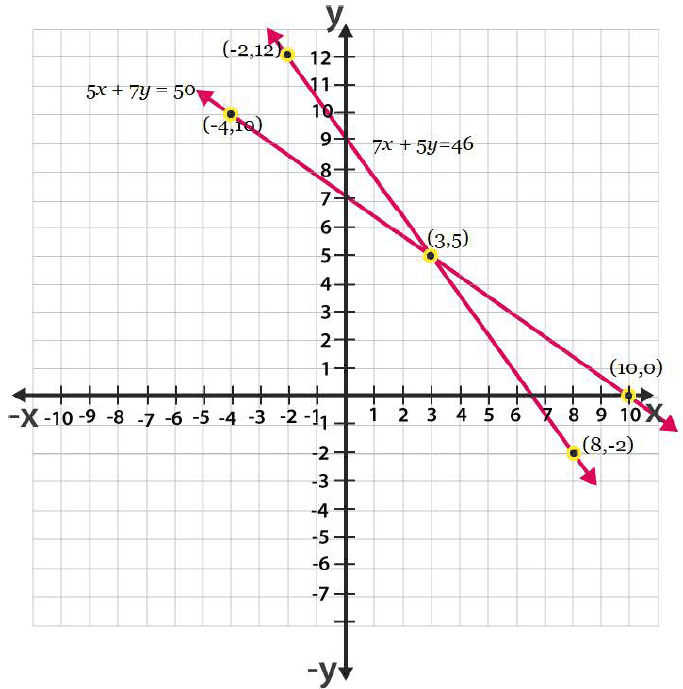For 2x + 2y = 10 or x = (10-2y)/2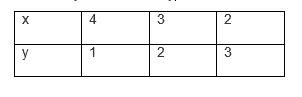So, the equations are represented in graphs as follows: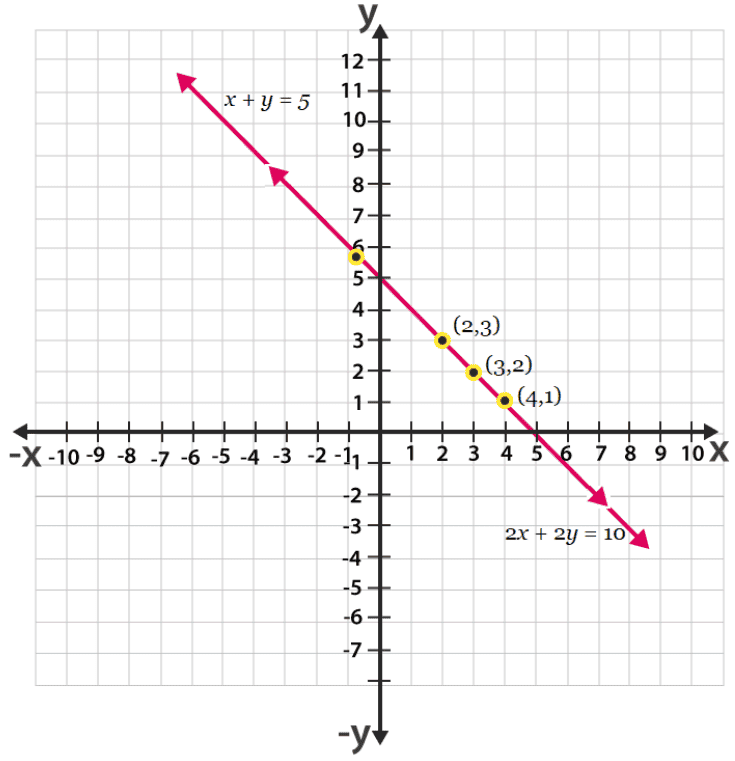From the figure, we can see, that the lines are overlapping each other.

Therefore, the equations have infinite possible solutions.

(ii) Given, x – y = 8 and 3x – 3y = 16

(a1/a2) = 1/3

(b1/b2) = -1/-3 = 1/3

(c1/c2) = 8/16 = 1/2

Since, (a1/a2) = (b1/b2) ≠ (c1/c2)

The equations are parallel to each other and have no solutions. Hence, the pair of linear equations is inconsistent.

(iii) Given, 2x + y – 6 = 0 and 4x – 2y – 4 = 0

(a1/a2) = 2/4 = ½

(b1/b2) = 1/-2

(c1/c2) = -6/-4 = 3/2

Since, (a1/a2) ≠ (b1/b2)

The given linear equations are intersecting each other at one point and have only one solution. Hence, the pair of linear equations is consistent.

Now, for2x + y – 6 = 0 or y = 6 – 2x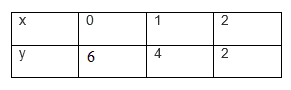And for4x – 2y – 4 = 0 or y = (4x-4)/2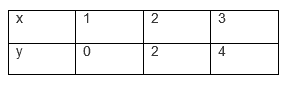So, the equations are represented in graphs as follows: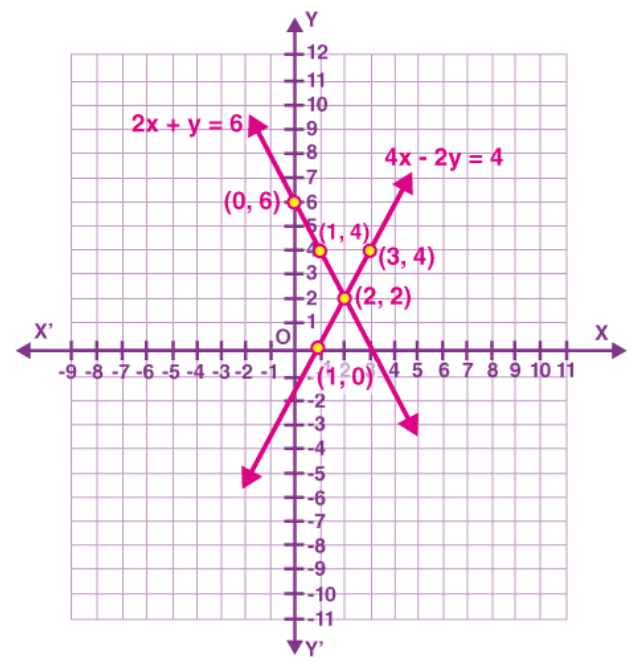From the graph, it can be seen that these lines are intersecting each other at only one point,(2,2).

(iv) Given, 2x – 2y – 2 = 0 and 4x – 4y – 5 = 0

(a1/a2) = 2/4 = ½

(b1/b2) = -2/-4 = 1/2

(c1/c2) = 2/5

Since, a1/a2 = b1/b2 ≠ c1/c2

Thus, these linear equations have parallel and have no possible solutions. Hence, the pair of linear equations are inconsistent.

5. Half the perimeter of a rectangular garden, whose length is 4 m more than its width, is 36 m. Find the dimensions of the garden.

Solutions:Let us consider.

The width of the garden is x and length is y.

Now, according to the question, we can express the given condition as;

y – x = 4

and

y + x = 36

Now, taking y – x = 4 or y = x + 4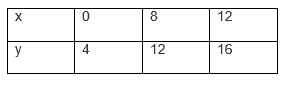For y + x = 36, y = 36 – x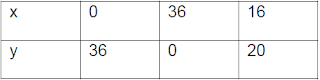The graphical representation of both the equation is as follows;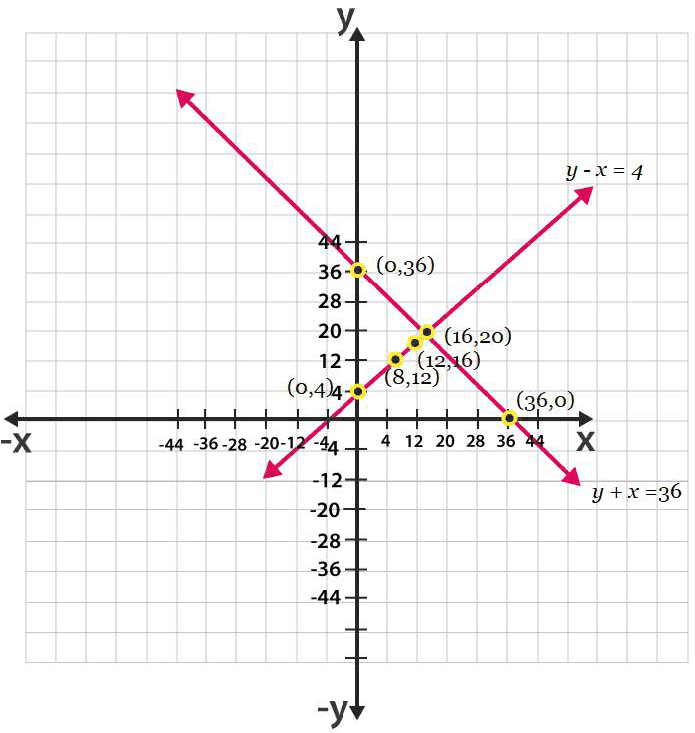From the graph you can see, the lines intersects each other at a point(16, 20). Hence, the width of the garden is 16 and length is 20.

6. Given the linear equation 2x + 3y – 8 = 0, write another linear equation in two variables such that the geometrical representation of the pair so formed is:

(i) Intersecting lines

(ii) Parallel lines

(iii) Coincident lines

Solutions:

(i) Given the linear equation 2x + 3y – 8 = 0.

To find another linear equation in two variables such that the geometrical representation of the pair so formed is intersecting lines, it should satisfy below condition;

(a1/a2) ≠ (b1/b2)

Thus, another equation could be 2x – 7y + 9 = 0, such that;

(a1/a2) = 2/2 = 1 and (b1/b2) = 3/-7

Clearly, you can see another equation satisfies the condition.

(ii) Given the linear equation 2x + 3y – 8 = 0.

To find another linear equation in two variables such that the geometrical representation of the pair so formed is parallel lines, it should satisfy below condition;

(a1/a2) = (b1/b2) ≠ (c1/c2)

Thus, another equation could be 6x + 9y + 9 = 0, such that;

(a1/a2) = 2/6 = 1/3

(b1/b2) = 3/9= 1/3

(c1/c2) = -8/9

Clearly, you can see another equation satisfies the condition.

(iii) Given the linear equation 2x + 3y – 8 = 0.

To find another linear equation in two variables such that the geometrical representation of the pair so formed is coincident lines, it should satisfy below condition;

(a1/a2) = (b1/b2) = (c1/c2)

Thus, another equation could be 4x + 6y – 16 = 0, such that;

(a1/a2) = 2/4 = 1/2 ,(b1/b2) = 3/6 = 1/2, (c1/c2) = -8/-16 = 1/2

Clearly, you can see another equation satisfies the condition.

7. Draw the graphs of the equations x – y + 1 = 0 and 3x + 2y – 12 = 0. Determine the coordinates of the vertices of the triangle formed by these lines and the x-axis, and shade the triangular region.

Solution: Given, the equations for graphs are x – y + 1 = 0 and 3x + 2y – 12 = 0.

For, x – y + 1 = 0 or x = -1+y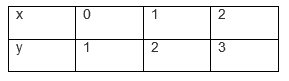For, 3x + 2y – 12 = 0 or x = (12-2y)/3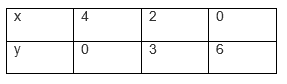Hence, the graphical representation of these equations is as follows;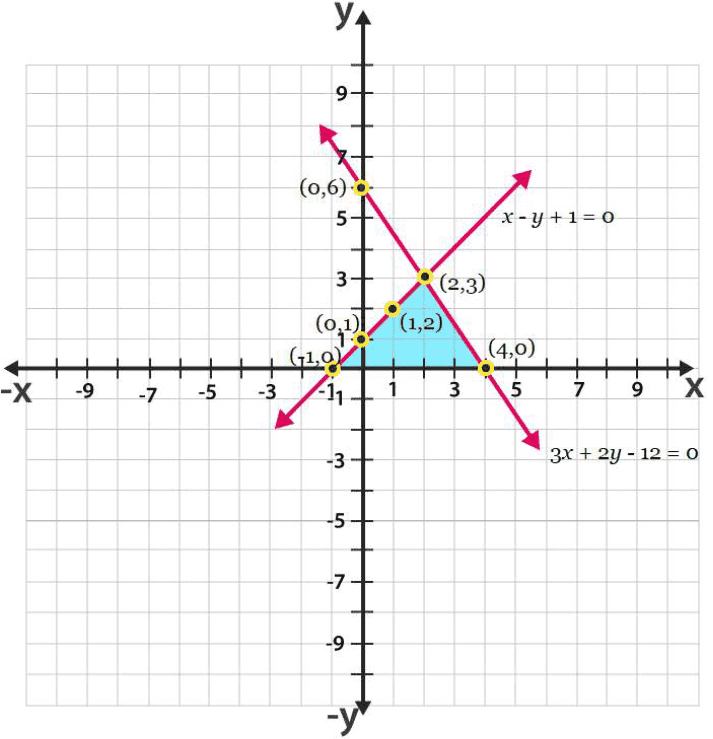From the figure, it can be seen that these lines are intersecting each other at point (2, 3) and x-axis at (−1, 0) and (4, 0). Therefore, the vertices of the triangle are (2, 3), (−1, 0), and (4, 0).

## Exercise 3.3 Page: 53

1. Solve the following pair of linear equations by the substitution method

(i) x + y = 14

x – y = 4

(ii) s – t = 3

(s/3) + (t/2) = 6

(iii) 3x – y = 3

9x – 3y = 9

(iv) 0.2x + 0.3y = 1.3

0.4x + 0.5y = 2.3

(v) √2 x+√3 y = 0

√3 x-√8 y = 0

(vi) (3x/2) – (5y/3) = -2

(x/3) + (y/2) = (13/6)

Solutions:

(i) Given,

x + y = 14 and x – y = 4 are the two equations.

From 1stequation, we get,

x = 14 – y

Now, substitute the value of x in second equation to get,

(14 – y) – y = 4

14 – 2y = 4

2y = 10

Or y = 5

By the value of y, we can now find the exact value of x;

∵ x = 14 – y

∴ x = 14 – 5

Or x = 9

Hence, x = 9 and y = 5.

(ii) Given,

s – t = 3 and (s/3) + (t/2) = 6 are the two equations.

From 1stequation, we get,

s = 3 + t ________________(1)

Now, substitute the value of s in second equation to get,

(3+t)/3 + (t/2) = 6

⇒ (2(3+t) + 3t )/6 = 6

⇒ (6+2t+3t)/6 = 6

⇒ (6+5t) = 36

⇒5t = 30

⇒t = 6

Now, substitute the value of t in equation (1)

s = 3 + 6 = 9

Therefore, s = 9 and t = 6.

(iii) Given,

3x – y = 3 and 9x – 3y = 9 are the two equations.

From 1stequation, we get,

x = (3+y)/3

Now, substitute the value of x in the given second equation to get,

9(3+y)/3 – 3y = 9

⇒9 +3y -3y = 9

⇒ 9 = 9

Therefore, y has infinite values and since, x = (3+y) /3, so x also has infinite values.

(iv) Given,

0.2x + 0.3y = 1.3 and 0.4x + 0.5y = 2.3are the two equations.

From 1stequation, we get,

x = (1.3- 0.3y)/0.2 _________________(1)

Now, substitute the value of x in the given second equation to get,

0.4(1.3-0.3y)/0.2 + 0.5y = 2.3

⇒2(1.3 – 0.3y) + 0.5y = 2.3

⇒ 2.6 – 0.6y + 0.5y = 2.3

⇒ 2.6 – 0.1 y = 2.3

⇒ 0.1 y = 0.3

⇒ y = 3

Now, substitute the value of y in equation (1), we get,

x = (1.3-0.3(3))/0.2 = (1.3-0.9)/0.2 = 0.4/0.2 = 2

Therefore, x = 2 and y = 3.

(v) Given,

√2 x + √3 y = 0 and √3 x – √8 y = 0

are the two equations.

From 1stequation, we get,

x = – (√3/√2)y __________________(1)

Putting the value of x in the given second equation to get,

√3(-√3/√2)y – √8y = 0 ⇒ (-3/√2)y- √8 y = 0

⇒ y = 0

Now, substitute the value of y in equation (1), we get,

x = 0

Therefore, x = 0 and y = 0.

(vi) Given,

(3x/2)-(5y/3) = -2 and (x/3) + (y/2) = 13/6 are the two equations.

From 1stequation, we get,

(3/2)x = -2 + (5y/3)

⇒ x = 2(-6+5y)/9 = (-12+10y)/9 ………………………(1)

Putting the value of x in the given second equation to get,

((-12+10y)/9)/3 + y/2 = 13/6

⇒y/2 = 13/6 –( (-12+10y)/27 ) + y/2 = 13/6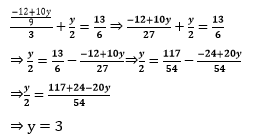Now, substitute the value of y in equation (1), we get,

(3x/2) – 5(3)/3 = -2

⇒ (3x/2) – 5 = -2

⇒ x = 2

Therefore, x = 2 and y = 3.

2. Solve 2x + 3y = 11 and 2x – 4y = – 24 and hence find the value of ‘m’ for which y = mx + 3.

Solution:

2x + 3y = 11…………………………..(I)

2x – 4y = -24………………………… (II)

From equation (II), we get

x = (11-3y)/2 ………………….(III)

Substituting the value of x in equation (II), we get

2(11-3y)/2 – 4y = 24

11 – 3y – 4y = -24

-7y = -35

y = 5……………………………………..(IV)

Putting the value of y in equation (III), we get

x = (11-3×5)/2 = -4/2 = -2

Hence, x = -2, y = 5

Also,

y = mx + 3

5 = -2m +3

-2m = 2

m = -1

Therefore the value of m is -1.

3. Form the pair of linear equations for the following problems and find their solution by substitution method.

(i) The difference between two numbers is 26 and one number is three times the other. Find them.

Solution:

Let the two numbers be x and y respectively, such that y > x.

According to the question,

y = 3x ……………… (1)

y – x = 26 …………..(2)

Substituting the value of (1) into (2), we get

3x – x = 26

x = 13 ……………. (3)

Substituting (3) in (1), we get y = 39

Hence, the numbers are 13 and 39.

(ii) The larger of two supplementary angles exceeds the smaller by 18 degrees. Find them.

Solution:

Let the larger angle by xo and smaller angle be yo.

We know that the sum of two supplementary pair of angles is always 180o.

According to the question,

x + y = 180o……………. (1)

x – y = 18……………..(2)

From (1), we get x = 180o – y …………. (3)

Substituting (3) in (2), we get

180– y – y =18o

162o = 2y

y = 81o ………….. (4)

Using the value of y in (3), we get

x = 180o – 81o

= 99o

Hence, the angles are 99o and 81o.

(iii) The coach of a cricket team buys 7 bats and 6 balls for Rs.3800. Later, she buys 3 bats and 5 balls for Rs.1750. Find the cost of each bat and each ball.

Solution:

Let the cost a bat be x and cost of a ball be y.

According to the question,

7x + 6y = 3800 ………………. (I)

3x + 5y = 1750 ………………. (II)

From (I), we get

y = (3800-7x)/6………………..(III)

Substituting (III) in (II). we get,

3x+5(3800-7x)/6 =1750

⇒3x+ 9500/3 – 35x/6 = 1750

⇒3x- 35x/6 = 1750 – 9500/3

⇒(18x-35x)/6 = (5250 – 9500)/3

⇒-17x/6 = -4250/3

⇒-17x = -8500

x = 500 ……………………….. (IV)

Substituting the value of x in (III), we get

y = (3800-7 ×500)/6 = 300/6 = 50

Hence, the cost of a bat is Rs 500 and cost of a ball is Rs 50.

(iv) The taxi charges in a city consist of a fixed charge together with the charge for the distance covered. For a distance of 10 km, the charge paid is Rs 105 and for a journey of 15 km, the charge paid is Rs 155. What are the fixed charges and the charge per km? How much does a person have to pay for travelling a distance of 25 km?

Solution:

Let the fixed charge be Rs x and per km charge be Rs y.

According to the question,

x + 10y = 105 …………….. (1)

x + 15y = 155 …………….. (2)

From (1), we get x = 105 – 10y ………………. (3)

Substituting the value of x in (2), we get

105 – 10y + 15y = 155

5y = 50

y = 10 …………….. (4)

Putting the value of y in (3), we get

x = 105 – 10 × 10 = 5

Hence, fixed charge is Rs 5 and per km charge = Rs 10

Charge for 25 km = x + 25y = 5 + 250 = Rs 255

(v) A fraction becomes 9/11 , if 2 is added to both the numerator and the denominator. If, 3 is added to both the numerator and the denominator it becomes 5/6. Find the fraction.

Solution:

Let the fraction be x/y.

According to the question,

(x+2) /(y+2) = 9/11

11x + 22 = 9y + 18

11x – 9y = -4 …………….. (1)

(x+3) /(y+3) = 5/6

6x + 18 = 5y +15

6x – 5y = -3 ………………. (2)

From (1), we get x = (-4+9y)/11 …………….. (3)

Substituting the value of x in (2), we get

6(-4+9y)/11 -5y = -3

-24 + 54y – 55y = -33

-y = -9

y = 9 ………………… (4)

Substituting the value of y in (3), we get

x = (-4+9×9 )/11 = 7

Hence the fraction is 7/9.

(vi) Five years hence, the age of Jacob will be three times that of his son. Five years ago, Jacob’s age was seven times that of his son. What are their present ages?

Solutions:

Let the age of Jacob and his son be x and y respectively.

According to the question,

(x + 5) = 3(y + 5)

x – 3y = 10 …………………………………….. (1)

(x – 5) = 7(y – 5)

x – 7y = -30 ………………………………………. (2)

From (1), we get x = 3y + 10 ……………………. (3)

Substituting the value of x in (2), we get

3y + 10 – 7y = -30

-4y = -40

y = 10 ………………… (4)

Substituting the value of y in (3), we get

x = 3 x 10 + 10 = 40

Hence, the present age of Jacob’s and his son is 40 years and 10 years respectively.

## Exercise 3.4 Page: 56

1. Solve the following pair of linear equations by the elimination method and the substitution method:

(i) x + y = 5 and 2x – 3y = 4

(ii) 3x + 4y = 10 and 2x – 2y = 2

(iii) 3x – 5y – 4 = 0 and 9x = 2y + 7

(iv) x/2+ 2y/3 = -1 and x-y/3 = 3

Solutions:

(i) x + y = 5 and 2x – 3y = 4

By the method of elimination.

x + y = 5 ……………………………….. (i)

2x – 3y = 4 ……………………………..(ii)

When the equation (i) is multiplied by 2, we get

2x + 2y = 10 ……………………………(iii)

When the equation (ii) is subtracted from (iii) we get,

5y = 6

y = 6/5 ………………………………………(iv)

Substituting the value of y in eq. (i) we get,

x=5−6/5 = 19/5

∴x = 19/5 , y = 6/5

By the method of substitution.

From the equation (i), we get:

x = 5 – y………………………………….. (v)

When the value is put in equation (ii) we get,

2(5 – y) – 3y = 4

-5y = -6

y = 6/5

When the values are substituted in equation (v), we get:

x =5− 6/5 = 19/5

∴x = 19/5 ,y = 6/5

(ii) 3x + 4y = 10 and 2x – 2y = 2

By the method of elimination.

3x + 4y = 10……………………….(i)

2x – 2y = 2 ………………………. (ii)

When the equation (i) and (ii) is multiplied by 2, we get:

4x – 4y = 4 ………………………..(iii)

When the Equation (i) and (iii) are added, we get:

7x = 14

x = 2 ……………………………….(iv)

Substituting equation (iv) in (i) we get,

6 + 4y = 10

4y = 4

y = 1

Hence, x = 2 and y = 1

By the method of Substitution

From equation (ii) we get,

x = 1 + y……………………………… (v)

Substituting equation (v) in equation (i) we get,

3(1 + y) + 4y = 10

7y = 7

y = 1

When y = 1 is substituted in equation (v) we get,

A = 1 + 1 = 2

Therefore, A = 2 and B = 1

(iii) 3x – 5y – 4 = 0 and 9x = 2y + 7

By the method of elimination:

3x – 5y – 4 = 0 ………………………………… (i)

9x = 2y + 7

9x – 2y – 7 = 0 …………………………………(ii)

When the equation (i) and (iii) is multiplied we get,

9x – 15y – 12 = 0 ………………………………(iii)

When the equation (iii) is subtracted from equation (ii) we get,

13y = -5

y = -5/13 ………………………………………….(iv)

When equation (iv) is substituted in equation (i) we get,

3x +25/13 −4=0

3x = 27/13

x =9/13

∴x = 9/13 and y = -5/13

By the method of Substitution:

From the equation (i) we get,

x = (5y+4)/3 …………………………………………… (v)

Putting the value (v) in equation (ii) we get,

9(5y+4)/3 −2y −7=0

13y = -5

y = -5/13

Substituting this value in equation (v) we get,

x = (5(-5/13)+4)/3

x = 9/13

∴x =9/13, y = -5/13

(iv) x/2 + 2y/3 = -1 and x-y/3 = 3

By the method of Elimination.

3x + 4y = -6 …………………………. (i)

x-y/3 = 3

3x – y = 9 ……………………………. (ii)

When the equation (ii) is subtracted from equation (i) we get,

5y = -15

y = -3 ………………………………….(iii)

When the equation (iii) is substituted in (i) we get,

3x – 12 = -6

3x = 6

x = 2

Hence, x = 2 , y = -3

By the method of Substitution:

From the equation (ii) we get,

x = (y+9)/3…………………………………(v)

Putting the value obtained from equation (v) in equation (i) we get,

3(y+9)/3 +4y =−6

5y = -15

y = -3

When y = -3 is substituted in equation (v) we get,

x = (-3+9)/3 = 2

Therefore, x = 2 and y = -3

2. Form the pair of linear equations in the following problems, and find their solutions (if they exist) by the elimination method:

(i) If we add 1 to the numerator and subtract 1 from the denominator, a fraction reduces to 1. It becomes if we only add 1 to the denominator. What is the fraction?

Solution:

Let the fraction be a/b

According to the given information,

(a+1)/(b-1) = 1

=> a – b = -2 ………………………………..(i)

a/(b+1) = 1/2

=> 2a-b = 1…………………………………(ii)

When equation (i) is subtracted from equation (ii) we get,

a = 3 …………………………………………………..(iii)

When a = 3 is substituted in equation (i) we get,

3 – b = -2

-b = -5

b = 5

Hence, the fraction is 3/5.

(ii) Five years ago, Nuri was thrice as old as Sonu. Ten years later, Nuri will be twice as old as Sonu. How old are Nuri and Sonu?

Solution:

Let us assume, present age of Nuri is x

And present age of Sonu is y.

According to the given condition, we can write as;

x – 5 = 3(y – 5)

x – 3y = -10…………………………………..(1)

Now,

x + 10 = 2(y +10)

x – 2y = 10…………………………………….(2)

Subtract eq. 1 from 2, to get,

y = 20 ………………………………………….(3)

Substituting the value of y in eq.1, we get,

x – 3.20 = -10

x – 60 = -10

x = 50

Therefore,

Age of Nuri is 50 years

Age of Sonu is 20 years.

(iii) The sum of the digits of a two-digit number is 9. Also, nine times this number is twice the number obtained by reversing the order of the digits. Find the number.

Solution:

Let the unit digit and tens digit of a number be x and y respectively.

Then, Number (n) = 10B + A

N after reversing order of the digits = 10A + B

According to the given information, A + B = 9…………………….(i)

9(10B + A) = 2(10A + B)

88 B – 11 A = 0

-A + 8B = 0 ………………………………………………………….. (ii)

Adding the equations (i) and (ii) we get,

9B = 9

B = 1……………………………………………………………………….(3)

Substituting this value of B, in the equation (i) we get A= 8

Hence the number (N) is 10B + A = 10 x 1 +8 = 18

(iv) Meena went to a bank to withdraw Rs.2000. She asked the cashier to give her Rs.50 and Rs.100 notes only. Meena got 25 notes in all. Find how many notes of Rs.50 and Rs.100 she received.

Solution:

Let the number of Rs.50 notes be A and the number of Rs.100 notes be B

According to the given information,

A + B = 25 ……………………………………………………………………….. (i)

50A + 100B = 2000 ………………………………………………………………(ii)

When equation (i) is multiplied with (ii) we get,

50A + 50B = 1250 …………………………………………………………………..(iii)

Subtracting the equation (iii) from the equation (ii) we get,

50B = 750

B = 15

Substituting in the equation (i) we get,

A = 10

Hence, Meena has 10 notes of Rs.50 and 15 notes of Rs.100.

(v) A lending library has a fixed charge for the first three days and an additional charge for each day thereafter. Saritha paid Rs.27 for a book kept for seven days, while Susy paid Rs.21 for the book she kept for five days. Find the fixed charge and the charge for each extra day.

Solution:

Let the fixed charge for the first three days be Rs.A and the charge for each day extra be Rs.B.

According to the information given,

A + 4B = 27 …………………………………….…………………………. (i)

A + 2B = 21 ……………………………………………………………….. (ii)

When equation (ii) is subtracted from equation (i) we get,

2B = 6

B = 3 …………………………………………………………………………(iii)

Substituting B = 3 in equation (i) we get,

A + 12 = 27

A = 15

Hence, the fixed charge is Rs.15

And the Charge per day is Rs.3

## Exercise 3.5 Page: 62

1. Which of the following pairs of linear equations has unique solution, no solution, or infinitely many solutions. In case there is a unique solution, find it by using cross multiplication method.

(i) x – 3y – 3 = 0 and 3x – 9y – 2 = 0 (ii) 2x + y = 5 and 3x + 2y = 8

(iii) 3x – 5y = 20 and 6x – 10y = 40 (iv) x – 3y – 7 = 0 and 3x – 3y – 15 = 0

Solutions:

(i) Given, x – 3y – 3 =0 and 3x – 9y -2 =0

a1/a2=1/3 ,         b1/b2= -3/-9 =1/3,     c1/c2=-3/-2 = 3/2

(a1/a2) = (b1/b2) ≠ (c1/c2)

Since, the given set of lines are parallel to each other they will not intersect each other and therefore there is no solution for these equations.

(ii) Given, 2x + y = 5 and 3x +2y = 8

a1/a2= 2/3 , b1/b2= 1/2 , c1/c2= -5/-8

(a1/a2) ≠ (b1/b2)

Since they intersect at a unique point these equations will have a unique solution by cross multiplication method:

x/(b1c2-c1b2) = y/(c1a2– c2a1) = 1/(a1b2-a2b1)

x/(-8-(-10)) = y/(-15-(-16)) = 1/(4-3)

x/2 = y/1 = 1

∴ x = 2 and y =1

(iii) Given, 3x – 5y = 20 and 6x – 10y = 40

(a1/a2) = 3/6 = 1/2

(b1/b2) = -5/-10 = 1/2

(c1/c2) = 20/40 = 1/2

a1/a2= b1/b2= c1/c2

Since the given sets of lines are overlapping each other there will be infinite number of solutions for this pair of equation.

(iv) Given, x – 3y – 7 = 0 and 3x – 3y – 15 = 0

(a1/a2) = 1/3

(b1/b2) = -3/-3 = 1

(c1/c2) = -7/-15

a1/a2≠ b1/b2

Since this pair of lines are intersecting each other at a unique point, there will be a unique solution.

By cross multiplication,

x/(45-21) = y/(-21+15) = 1/(-3+9)

x/24 = y/ -6 = 1/6

x/24 = 1/6 and y/-6 = 1/6

∴ x = 4 and y = 1.

2. (i) For which values of a and b does the following pair of linear equations have an infinite number of solutions?

2x + 3y = 7

(a – b) x + (a + b) y = 3a + b – 2

(ii) For which value of k will the following pair of linear equations have no solution?

3x + y = 1

(2k – 1) x + (k – 1) y = 2k + 1

Solution:

(i) 3y + 2x -7 =0

(a + b)y + (a-b)y – (3a + b -2) = 0

a1/a2= 2/(a-b) ,               b1/b2= 3/(a+b) ,               c1/c2= -7/-(3a + b -2)

For infinitely many solutions,

a1/a2= b1/b2= c1/c2

Thus 2/(a-b) = 7/(3a+b– 2)

6a + 2b – 4 = 7a – 7b

a – 9b = -4  ……………………………….(i)

2/(a-b) = 3/(a+b)

2a + 2b = 3a – 3b

a – 5b = 0 ……………………………….….(ii)

Subtracting (i) from (ii), we get

4b = 4

b =1

Substituting this eq. in (ii), we get

a -5 x 1= 0

a = 5

Thus at a = 5 and b = 1 the given equations will have infinite solutions.

(ii) 3x + y -1 = 0

(2k -1)x  +  (k-1)y – 2k -1 = 0

a1/a2= 3/(2k -1) ,           b1/b2= 1/(k-1), c1/c2 = -1/(-2k -1) = 1/( 2k +1)

For no solutions

a1/a2= b1/b2≠ c1/c2

3/(2k-1) = 1/(k -1)   ≠ 1/(2k +1)

3/(2k –1) = 1/(k -1)

3k -3 = 2k -1

k =2

Therefore, for k = 2 the given pair of linear equations will have no solution.

3. Solve the following pair of linear equations by the substitution and cross-multiplication methods:

8x + 5y = 9

3x + 2y = 4

Solution:

8x + 5y = 9 …………………..(1)

3x + 2y = 4 ……………….….(2)

From equation (2) we get

x = (4 – 2y )/ 3  ……………………. (3)

Using this value in equation 1, we get

8(4-2y)/3 + 5y = 9

32 – 16y +15y = 27

-y = -5

y = 5 ……………………………….(4)

Using this value in equation (2), we get

3x + 10 = 4

x = -2

Thus, x = -2 and y = 5.

Now, Using Cross Multiplication method:

8x +5y – 9 = 0

3x + 2y – 4 = 0

x/(-20+18) = y/(-27 + 32 ) = 1/(16-15)

-x/2 = y/5 =1/1

∴ x = -2 and y =5.

4. Form the pair of linear equations in the following problems and find their solutions (if they exist) by any algebraic method:

(i) A part of monthly hostel charges is fixed and the remaining depends on the number of days one has taken food in the mess. When a student A takes food for 20 days she has to pay Rs.1000 as hostel charges whereas a student B, who takes food for 26 days, pays Rs.1180 as hostel charges. Find the fixed charges and the cost of food per day.

(ii) A fraction becomes 1/3 when 1 is subtracted from the numerator and it becomes 1/4 when 8 is added to its denominator. Find the fraction.

(iii) Yash scored 40 marks in a test, getting 3 marks for each right answer and losing 1 mark for each wrong answer. Had 4 marks been awarded for each correct answer and 2 marks been deducted for each incorrect answer, then Yash would have scored 50 marks. How many questions were there in the test?

(iv) Places A and B are 100 km apart on a highway. One car starts from A and another from B at the same time. If the cars travel in the same direction at different speeds, they meet in 5 hours. If they travel towards each other, they meet in 1 hour. What are the speeds of the two cars?

(v) The area of a rectangle gets reduced by 9 square units, if its length is reduced by 5 units and breadth is increased by 3 units. If we increase the length by 3 units and the breadth by 2 units, the area increases by 67 square units. Find the dimensions of the rectangle.

Solutions:

(i)Let x be the fixed charge and y be the charge of food per day.

According to the question,

x + 20y = 1000……………….. (i)

x + 26y = 1180………………..(ii)

Subtracting (i) from  (ii) we get

6y = 180

y = Rs.30

Using this value in equation (ii) we get

x = 1180 -26 x 30

x= Rs.400.

Therefore, fixed charges is Rs.400 and charge per day is Rs.30.

(ii) Letthe fraction be x/y.

So, as per the question given,

(x -1)/y = 1/3 => 3x – y = 3…………………(1)

x/(y + 8) = 1/4  => 4x –y =8 ………………..(2)

Subtracting equation (1) from (2) , we get

x = 5 ………………………………………….(3)

Using this value in equation (2), we get,

(4×5)– y = 8

y= 12

Therefore, the fraction is 5/12.

(iii) Let the number of right answers is x and number of wrong answers be y

According to the given question;

3x−y=40……..(1)

4x−2y=50

⇒2x−y=25…….(2)

Subtracting equation (2) from equation (1), we get;

x = 15 ….….(3)

Putting this in equation (2), we obtain;

30 – y = 25

Or y = 5

Therefore, number of right answers = 15 and number of wrong answers = 5

Hence, total number of questions = 20

(iv) Let x km/h be the speed of car from point A and y km/h be the speed of car from point B.

If the car travels in the same direction,

5x – 5y = 100

x – y = 20 …………………………………(i)

If the car travels in the opposite direction,

x + y = 100………………………………(ii)

Solving equation (i) and (ii), we get

x = 60 km/h………………………………………(iii)

Using this in equation (i), we get,

60 – y = 20

y = 40 km/h

Therefore, the speed of car from point A = 60 km/h

Speed of car from point B = 40 km/h.

(v) Let,

The length of rectangle = x unit

And breadth of the rectangle = y unit

Now, as per the question given,

(x – 5) (y + 3) = xy -9

3x – 5y – 6 = 0……………………………(1)

(x + 3) (y + 2) = xy + 67

2x + 3y – 61 = 0…………………………..(2)

Using cross multiplication method, we get,

x/(305 +18) = y/(-12+183) = 1/(9+10)

x/323 = y/171 = 1/19

Therefore, x = 17 and y = 9.

Hence, the length of rectangle = 17 units

And breadth of the rectangle = 9 units

## Exercise 3.6 Page: 67

1. Solve the following pairs of equations by reducing them to a pair of linear equations:

(i) 1/2x + 1/3y = 2

1/3x + 1/2y = 13/6

Solution:

Let us assume 1/x = m and 1/y = n  , then the equation will change as follows.

m/2 + n/3 = 2

⇒ 3m+2n-12 = 0…………………….(1)

m/3 + n/2 = 13/6

⇒ 2m+3n-13 = 0……………………….(2)

Now, using cross-multiplication method, we get,

m/(-26-(-36) ) = n/(-24-(-39)) = 1/(9-4)

m/10 = n/15 = 1/5

m/10 = 1/5 and n/15 = 1/5

So, m = 2 and n = 3

1/x = 2 and 1/y = 3

x = 1/2 and y = 1/3

(ii) 2/√x + 3/√y = 2

4/√x + 9/√y = -1

Solution:

Substituting 1/√x = m and 1/√y = n in the given equations, we get

2m + 3n = 2 ………………………..(i)

4m – 9n = -1 ………………………(ii)

Multiplying equation (i) by 3, we get

6m + 9n = 6 ………………….…..(iii)

Adding equation (ii) and (iii), we get

10m = 5

m = 1/2…………………………….…(iv)

Now by putting the value of ‘m’ in equation (i), we get

2×1/2 + 3n = 2

3n = 1

n = 1/3

m =1/√x

½ = 1/√x

x = 4

n = 1/√y

1/3 = 1/√y

y = 9

Hence, x = 4 and y = 9

(iii) 4/x + 3y = 14

3/x -4y = 23

Solution:

Putting in the given equation we get,

So, 4m + 3y = 14     => 4m + 3y – 14 = 0  ……………..…..(1)

3m – 4y = 23     => 3m – 4y – 23 = 0  ……………………….(2)

By cross-multiplication, we get,

m/(-69-56) = y/(-42-(-92)) = 1/(-16-9)

-m/125 = y/50 = -1/ 25

-m/125 = -1/25 and y/50 = -1/25

m = 5 and b = -2

m = 1/x = 5

So , x = 1/5

y = -2

(iv) 5/(x-1) + 1/(y-2) = 2

6/(x-1) – 3/(y-2) = 1

Solution:

Substituting 1/(x-1) = m and 1/(y-2) = n  in the given equations, we get,

5m + n = 2 …………………………(i)

6m – 3n = 1 ……………………….(ii)

Multiplying equation (i) by 3, we get

15m + 3n = 6 …………………….(iii)

Adding (ii) and (iii) we get

21m = 7

m = 1/3

Putting this value in equation (i), we get

5×1/3 + n = 2

n = 2- 5/3 = 1/3

m = 1/ (x-1)

⇒ 1/3 = 1/(x-1)

⇒ x = 4

n = 1/(y-2)

⇒ 1/3 = 1/(y-2)

⇒ y = 5

Hence, x = 4 and y = 5

(v) (7x-2y)/ xy = 5

(8x + 7y)/xy = 15

Solution:

(7x-2y)/ xy = 5

7/y – 2/x = 5…………………………..(i)

(8x + 7y)/xy = 15

8/y + 7/x = 15…………………………(ii)

Substituting 1/x =m in the given equation we get,

– 2m + 7n = 5     => -2 + 7n – 5 = 0  ……..(iii)

7m + 8n = 15     => 7m + 8n – 15 = 0 ……(iv)

By cross-multiplication method, we get,

m/(-105-(-40)) = n/(-35-30) = 1/(-16-49)

m/(-65) = n/(-65) = 1/(-65)

m/-65 = 1/-65

m = 1

n/(-65) = 1/(-65)

n = 1

m = 1 and n = 1

m = 1/x = 1        n = 1/x = 1

Therefore, x = 1 and y = 1

(vi) 6x + 3y = 6xy

2x + 4y = 5xy

Solution:

6x + 3y = 6xy

6/y + 3/x = 6

Let 1/x = m and 1/y = n

=> 6n +3m = 6

=>3m + 6n-6 = 0…………………….(i)

2x + 4y = 5xy

=> 2/y + 4/x = 5

=> 2n +4m = 5

=> 4m+2n-5 = 0……………………..(ii)

3m + 6n – 6 = 0

4m + 2n – 5 = 0

By cross-multiplication method, we get

m/(-30 –(-12)) = n/(-24-(-15)) = 1/(6-24)

m/-18 = n/-9 = 1/-18

m/-18 = 1/-18

m = 1

n/-9 = 1/-18

n = 1/2

m = 1 and n = 1/2

m = 1/x = 1 and n = 1/y = 1/2

x = 1 and y = 2

Hence, x = 1 and y = 2

(vii) 10/(x+y) + 2/(x-y) = 4

15/(x+y) – 5/(x-y) = -2

Solution:

Substituting 1/x+y = m and 1/x-y = n in the given equations, we get,

10m + 2n = 4      =>  10m + 2n – 4 = 0      ………………..…..(i)

15m – 5n = -2     =>   15m – 5n + 2 = 0    ……………………..(ii)

Using cross-multiplication method, we get,

m/(4-20) = n/(-60-(20)) = 1/(-50 -30)

m/-16 = n/-80 = 1/-80

m/-16 = 1/-80 and n/-80 = 1/-80

m = 1/5 and n = 1

m = 1/(x+y) = 1/5

x+y = 5 …………………………………………(iii)

n = 1/(x-y) = 1

x-y = 1……………………………………………(iv)

Adding equation (iii) and (iv), we get

2x = 6   => x = 3 …….(v)

Putting the value of x = 3 in equation (3), we get

y = 2

Hence, x = 3 and y = 2

(viii) 1/(3x+y) + 1/(3x-y) = 3/4

1/2(3x+y) – 1/2(3x-y) = -1/8

Solution:

Substituting 1/(3x+y) = m and 1/(3x-y) = n in the given equations, we get,

m + n = 3/4 …………………………….…… (1)

m/2 – n/2 = -1/8

m – n = -1/4  …………………………..…(2)

Adding (1) and (2), we get

2m = 3/4 – 1/4

2m = 1/2

Putting in (2), we get

1/4 – n = -1/4

n = 1/4 + 1/4 = 1/2

m = 1/(3x+y) = 1/4

3x + y = 4  …………………………………(3)

n = 1/( 3x-y) = 1/2

3x – y = 2 ………………………………(4)

Adding equations (3) and (4), we get

6x = 6

x = 1 ……………………………….(5)

Putting in (3), we get

3(1) + y = 4

y = 1

Hence, x = 1 and y = 1

2. Formulate the following problems as a pair of equations, and hence find their solutions:

(i) Ritu can row downstream 20 km in 2 hours, and upstream 4 km in 2 hours. Find her speed of rowing in still water and the speed of the current.

(ii) 2 women and 5 men can together finish an embroidery work in 4 days, while 3 women and 6 men can finish it in 3 days. Find the time taken by 1 woman alone to finish the work, and also that taken by 1 man alone.

(iii) Roohi travels 300 km to her home partly by train and partly by bus. She takes 4 hours if she travels 60 km by train and the remaining by bus. If she travels 100 km by train and the remaining by bus, she takes 10 minutes longer. Find the speed of the train and the bus separately.

Solutions:

(i) Let us consider,

Speed of Ritu in still water = x km/hr

Speed of Stream = y km/hr

Now, speed of Ritu during,

Downstream = x + y km/h

Upstream = x – y km/h

As per the question given,

2(x+y) = 20

Or x + y = 10……………………….(1)

And, 2(x-y) = 4

Or x – y = 2………………………(2)

Adding both the eq.1 and 2, we get,

2x = 12

x = 6

Putting the value of x in eq.1, we get,

y = 4

Therefore,

Speed of Ritu rowing in still water = 6 km/hr

Speed of Stream = 4 km/hr

(ii) Let us consider,

Number of days taken by women to finish the work = x

Number of days taken by men to finish the work = y

Work done by women in one day = 1/x

Work done by women in one day = 1/y

As per the question given,

4(2/x + 5/y) = 1

(2/x + 5/y) = 1/4

And, 3(3/x + 6/y) = 1

(3/x + 6/y) = 1/3

Now, put 1/x=m and 1/y=n, we get,

2m + 5n = 1/4 => 8m + 20n = 1…………………(1)

3m + 6n =1/3 => 9m + 18n = 1………………….(2)

Now, by cross multiplication method, we get here,

m/(20-18) = n/(9-8) = 1/ (180-144)

m/2 = n/1 = 1/36

m/2 = 1/36

m = 1/18

m = 1/x = 1/18

or x = 18

n = 1/y = 1/36

y = 36

Therefore,

Number of days taken by women to finish the work = 18

Number of days taken by men to finish the work = 36.

(iii) Let us consider,

Speed of the train = x km/h

Speed of the bus = y km/h

According to the given question,

60/x + 240/y = 4 …………………(1)

100/x + 200/y = 25/6 …………….(2)

Put 1/x=m and 1/y=n, in the above two equations;

60m + 240n = 4……………………..(3)

100m + 200n = 25/6

600m + 1200n = 25 ………………….(4)

Multiply eq.3 by 10, to get,

600m + 2400n = 40 ……………………(5)

Now, subtract eq.4 from 5, to get,

1200n = 15

n = 15/1200 = 1/80

Substitute the value of n in eq. 3, to get,

60m + 3 = 4

m = 1/60

m = 1/x = 1/60

x = 60

And y = 1/n

y = 80

Therefore,

Speed of the train = 60 km/h

Speed of the bus = 80 km/h

## Exercise 3.7 Page: 68

1. The ages of two friends Ani and Biju differ by 3 years. Ani’s father Dharam is twice as old as Ani and Biju is twice as old as his sister Cathy. The ages of Cathy and Dharam differ by 30 years. Find the ages of Ani and Biju.

Solution:

The age difference between Ani and Biju is 3 yrs.

Either Biju is 3 years older than that of Ani or Ani is 3 years older than Biju. From both the cases we find out that Ani’s father’s age is 30 yrs more than that of Cathy’s age.

Let the ages of Ani and Biju be A and B respectively.

Therefore, the age of Dharam = 2 x A = 2A yrs.

And the age of Biju sister Ann B/2 yrs

By using the information that is given,

Case (i)

When Ani is older than that of Biju by 3 yrs then A – B = 3 – – – – – – – – (1)

2A−B/2 = 30

4A – B = 60 – – – – – – – – – – – (2)

By subtracting the equations (1) and (2) we get,

3A = 60 – 3 = 57

A = 57/3 = 19

Therefore, the age of Ani = 19 yrs

And the age of Biju is 19 – 3 = 16 yrs.

Case (ii)

When Biju is older than Ani,

B – A = 3 – – – – – – – – – (1)

2A − B/2 = 30

4A – B = 60 – – – – – – – – – (2)

Adding the equation (1) and (2) we get,

3A = 63

A = 21

Therefore, the age of Ani is 21 yrs

And the age of Biju is 21 + 3 = 24 yrs.

2. One says, “Give me a hundred, friend! I shall then become twice as rich as you”. The other replies, “If you give me ten, I shall be six times as rich as you”. Tell me what is the amount of their (respective) capital? [From the Bijaganita of Bhaskara II] [Hint : x + 100 = 2(y – 100), y + 10 = 6(x – 10)].

Solution:

Let Sangam have Rs A with him and Reuben have Rs B with him.

Using the information that is given we get,

A + 100 = 2(B – 100) ⇒ A + 100 = 2B – 200

Or A – 2B = -300 – – – – – – – (1)

And

6(A – 10) = ( B + 10 )

Or 6A – 60 = B + 10

Or 6A – B = 70 – – – – – – (2)

When equation (2) is multiplied by 2 we get,

12A – 2B = 140 – – – – – – – (3)

When equation (1) is subtracted from equation (3) we get,

11A = 140 + 300

11A = 440

⇒ A = 440/11 = 40

Using A =40 in equation (1) we get,

40 – 2B = -300

40 + 300 = 2B

2B = 340

B = 170

Therefore, Sangam had Rs 40 and Reuben had Rs 170 with them.

3. A train covered a certain distance at a uniform speed. If the train would have been 10 km/h faster, it would have taken 2 hours less than the scheduled time. And, if the train were slower by 10 km/h; it would have taken 3 hours more than the scheduled time. Find the distance covered by the train.

Solution:

Let the speed of the train be A km/hr and the time taken by the train to travel a distance be N hours and the distance to travel be X hours.

Speed of the train = Distance travelled by train / Time taken to travel that distance

A = N (distance)/ X (time)

Or, N = AX – – – – – – – – – – – (1)

Using the information that is given, we get:

(A+10) = X/(N-2)

(A + 10) (N – 2) = X

AN + 10N – 2A – 20 = X

By using the equation (1) we get,

– 2A + 10N = 20 – – – – – – – – – – (2)

(A-10) = X/(N+3)

(A – 10) (N + 3) = X

AN – 10N + 3A – 30 = X

By using the equation (1) we get,

3A – 10N = 30 – – – – – – – – – (3)

Adding equation (2) and equation (3) we get,

A = 50

Using the equation (2) we get,

(-2) x (50) + 10N = 20

-100 +10N = 20

=> 10N = 120

N = 12hours

From the equation (1) we get,

Distance travelled by the train, X = AN

= 50 x 12

= 600 km

Hence, the distance covered by the train is 600km.

4. The students of a class are made to stand in rows. If 3 students are extra in a row, there would be 1 row less. If 3 students are less in a row, there would be 2 rows more. Find the number of students in the class.

Solution:

Let the number of rows be A and the number of students in a row be B.

Total number of students = Number of rows x Number of students in a row

=AB

Using the information, that is given,

First Condition:

Total number of students = (A – 1) ( B + 3)

Or AB = ( A – 1 )(B + 3) = AB – B + 3A – 3

Or 3A – B – 3 = 0

Or 3A – Y = 3 – – – – – – – – – – – – – (1)

Second condition:

Total Number of students = (A + 2 ) ( B – 3 )

Or AB = AB + 2B – 3A – 6

Or 3A – 2B = -6 – – – – – – – – – (2)

When equation (2) is subtracted from (1)

(3A – B) – (3A – 2B) = 3 – (-6)

-B + 2B = 3 + 6B = 9

By using the equation (1) we get,

3A – 9 =3

3A = 9+3 = 12

A = 4

Number of rows, A = 4

Number of students in a row, B = 9

Number of total students in a class = AB = 4 x 9 = 36

5. In a ∆ABC, ∠ C = 3 ∠ B = 2 (∠A + ∠ B). Find the three angles.

Solution:

Given,

∠C = 3 ∠B = 2(∠B + ∠A)

3∠B = 2 ∠A+2 ∠B

∠B=2 ∠A

2∠A – ∠B= 0- – – – – – – – – – – –  (i)

We know, the sum of all the interior angles of a triangle is 180O.

Thus, ∠ A +∠B+ ∠C = 180O

∠A + ∠B +3 ∠B = 180O

∠A + 4 ∠B = 180O– – – – – – – – – – – – – – -(ii)

Multiplying 4 to  equation (i) , we get

8 ∠A – 4 ∠B = 0- – – – – – – – – – – – (iii)

Adding equations (iii) and (ii) we get

9 ∠A = 180O

∠A = 20O

Using this in equation (ii), we get

20O+ 4∠B = 180O

∠B = 40O

3∠B =∠C

∠C = 3 x 40 = 120O

Therefore, ∠A = 20O

∠B=40O

∠C = 120O

6. Draw the graphs of the equations 5x – y = 5 and 3x – y = 3. Determine the co-ordinates of the vertices of the triangle formed by these lines and the y axis.

Solutions:

Given,

5x – y = 5

=> y = 5x – 5

Its solution table will be.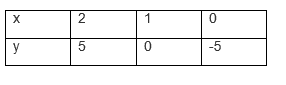Also given,3x – y = 3

y = 3x – 3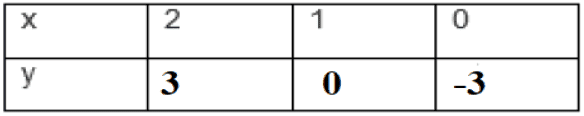The graphical representation of these lines will be as follows: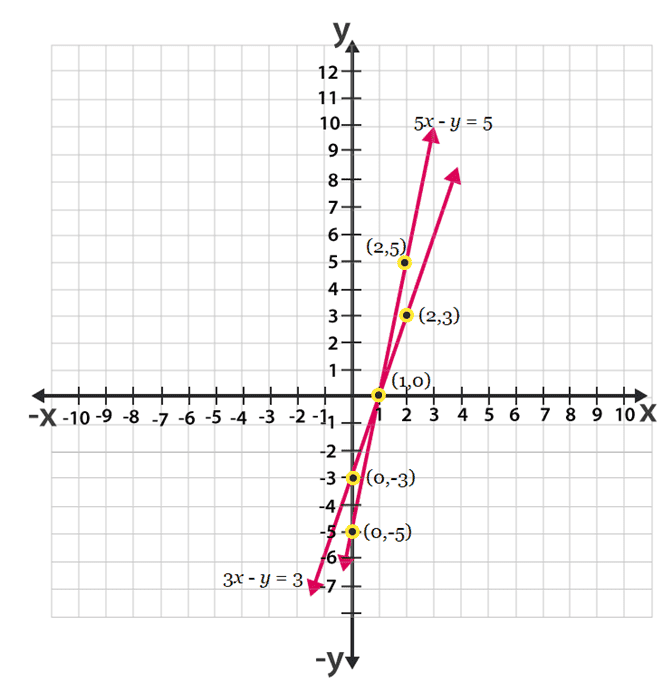From the above graph we can see that the triangle formed is ∆ABC by the lines and the y axis. Also the coordinates of the vertices are A(1,0) ,  C(0,-5) and B(0,-3).

7. Solve the following pair of linear equations:

(i) px + qy = p – q

qx – py = p + q

(ii) ax + by = c

bx + ay = 1 + c

(iii) x/a – y/b = 0

ax + by = a2+ b2

(iv) (a – b)x + (a + b) y = a2– 2ab – b2

(a + b)(x + y) = a2+ b2

(v) 152x – 378y = – 74

–378x + 152y = – 604

Solutions:

(i) px + qy = p – q……………(i)

qx – py = p + q……………….(ii)

Multiplying p to equation (1)  and q to equation (2), we get

p2x + pqy = p2 − pq ………… (iii)

q2x − pqy = pq + q2 ………… (iv)

Adding equation (iii) and equation (iv),we get

p2x + q2 x = p2  + q2

(p2 + q2 ) x = p2 + q2

x = (p2+ q2)/ p2 + q2= 1

From equation (i), we get

p(1) + qy = p – q

qy = p-q-p

qy = -q

y = -1

(ii) ax + by= c…………………(i)

bx + ay = 1+ c………… ..(ii)

Multiplying a to equation (i) and  b to equation (ii), we obtain

a2x + aby = ac ………………… (iii)

b2x + aby = b + bc…………… (iv)

Subtracting equation (iv) from equation (iii),

(a2– b2) x = ac − bc– b

x = (ac − bc– b)/ (a2– b2)

x = c(a-b) –b / (a2+b2)

From equation (i), we obtain

ax +by = c

a{c(a−b)−b)/ (a2– b2)} +by=c

ac(a−b)−ab/ (a2– b2)+by=c

by=c–ac(a−b)−ab/(a2– b2)

by=abc – b2c+ab/a2-b2

y = c(a-b)+a/a2-b2

(iii) x/a – y/b = 0

ax + by = a2+ b2

x/a – y/b = 0

=> bx − ay = 0 ……. (i)

ax + by = a2+ b2…….. (ii)

Multiplying a and b to equation (i) and (ii) respectively, we get

b2x − aby = 0 …………… (iii)

a2x + aby = a 3 + ab3 …… (iv)

Adding equations (iii) and (iv), we get

b2x + a2x = a 3 + ab2

x (b2 + a2) = a (a2 + b2) x = a

Using equation (i), we get

b(a) − ay = 0

ab − ay = 0

ay = ab,

y = b

(iv) (a – b)x + (a + b) y = a2– 2ab – b2

(a + b)(x + y) = a2+ b2

(a + b) y + (a – b) x = a2− 2ab − b2 …………… (i)

(x + y)(a + b)  = a 2 + b2

(a + b) y + (a + b) x  = a2+ b2………………… (ii)

Subtracting equation (ii) from equation (i), we get

(a − b) x − (a + b) x = (a 2 − 2ab − b 2) − (a2 + b2)

x(a − b − a − b) = − 2ab − 2b2

− 2bx = − 2b (b + a)

x = b + a

Substituting this value in equation (i), we get

(a + b)(a − b)  +y (a + b)  = a2− 2ab – b2

a2 − b2 + y(a + b)  = a2− 2ab – b2

(a + b) y = − 2ab

y = -2ab/(a+b)

(v) 152x − 378y = − 74

76x − 189y = − 37

x =(189y-37)/76……………..…(i)

− 378x + 152y = − 604

− 189x + 76y = − 302 ………….. (ii)

Using the value of x in equation (ii), we get

−189(189y−37)/76+76y=−302

− (189)2y + 189 × 37 + (76)2 y = − 302 × 76

189 × 37 + 302 × 76 = (189)2 y − (76)2y

6993 + 22952 = (189 − 76) (189 + 76) y

29945 = (113) (265) y

y = 1

Using equation (i), we get

x = (189-37)/76

x = 152/76 = 2

8. ABCD is a cyclic quadrilateral (see Fig. 3.7). Find the angles of the cyclic quadrilateral.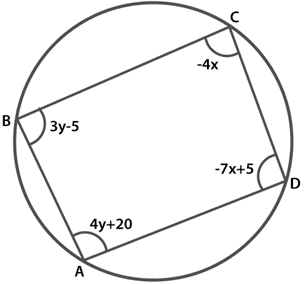Solution:

It is known that the sum of the opposite angles of a cyclic quadrilateral is 180o

Thus, we have

∠C +∠A = 180

4y + 20− 4x = 180

− 4x + 4y = 160

x − y = − 40 ……………(1)

And, ∠B + ∠D = 180

3y − 5 − 7x + 5 = 180

− 7x + 3y = 180 ………..(2)

Multiplying 3 to equation (1), we get

3x − 3y = − 120 ………(3)

Adding equation (2) to equation (3), we get

− 7x + 3x = 180 – 120

− 4x = 60

x = −15

Substituting this value in equation (i), we get

x − y = − 40

-y−15 = − 40

y = 40-15

= 25

∠A = 4y + 20 = 20+4(25) = 120°

∠B = 3y − 5 = − 5+3(25) = 70°

∠C = − 4x = − 4(− 15) = 60°

∠D = 5-7x

∠D= 5− 7(−15) = 110°

Hence, all the angles are measured.